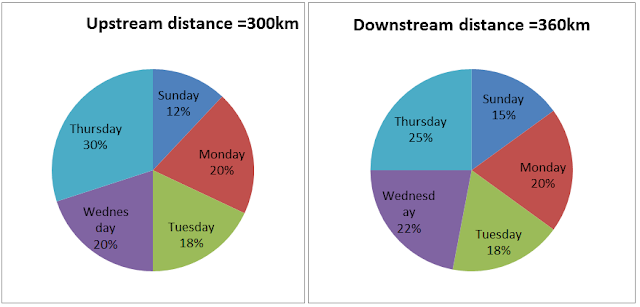# Data Interepretation Practice Set: Part 15

Directions- The following pie chart shows the distance travelled by boat on different days. The speed of the stream is same in both upstream and downstream direction. The table shows the speed of stream at different days. Study the data given carefully and answer the questions based on it.Days Speed of the stream (km/hr) Sunday 2 Monday 3 Tuesday 2.5 Wednesday - Thursday 2

1. On Wednesday the difference between speeds of the boat going downstream and upstream is 7km/hr and also the ratio of the speed of boat going downstream and upstream 11: 4. If the total time taken by boat to cover downstream and upstream distances on Wednesday is 26 hours 36 minutes. Find the speed of stream on that day.
a. 1
b. 1.5
c. 2
d. 2.5
e. None of the above

2. If the total speed of a boat in still water on Sunday and Tuesday is 12.5 km/hr and also time taken to cover the downstream distance on Sunday is equal to the time taken to cover the upstream distance on Tuesday. Find the ratio of speed in still water on Sunday and Tuesday.
a. 9:16
b. 16:17
c. 16:9
d. 19:16
e. None of the above

3. On Thursday the boat takes total 30 hours to cover upstream and downstream distances. If the ratio of the speed of the boat in still water going downstream and upstream is 5:3. Find the speed of the boat in still water while going in an upstream direction.
a. 8km/hr
b. 12km/hr
c. 6 km/hr
d. 19km/hr
e. None of the above
4. Time taken to cover the upstream distance on Monday is 8 hours more than the time taken to cover the downstream distance on Thursday and total speed in still water on Monday and Thursday is 23 km/hr. Find the ratio of speed in still water on Monday and Thursday.
a. 5:18
b. 7:16
c. 10:13
d. 11:12
e. None of the above
5. On Sunday the ratio of the speed of the boat in still water going upstream and downstream is 5:7. The difference between the time to cover upstream and downstream distance is 6 hours. Find the total time to cover the upstream and downstream distance.

a. 6 hours
b. 12hours
c. 18hours
d. 15hours
e. None of the above

## 1. Solution

let speed of stream = v
Ratio of upstream: ratio of downstream= 4:11
Difference = 7km/hr
So upstream speed = 4
downstream speed=11
Now
{22% of 360/ (11+v) + 20%of 300/(4-v)}= 26.6
On solving we get v= 1km/hr
So option 1 is correct.

## 2. Solution

Solution- let speed of boat on Sunday =u
Speed of boat on Tuesday = v
(u + v)=12.5km/hr
According to question
(v+2)/ (u-2.5) = 15% of 360/18%of 300
(Time same so ratio of speed = ratio of dist.)
V+2= u-2.5
u -v= 4.5
u=8, v=4.5
Therefore u: v = 16:9
Option 3 is correct.

## 3. Solution

(25%of 360)/ (5x+2) + (30%of 300)/ (3x-2) = 30
90/ (5x+2) +90/ (3x-2) = 30
X=2
Hence speed in upstream = 3 * 2 = 6km/hr
Option 3 is correct.

## 4. Solution

{20%of300/ (u1-2) -20%of 360/ (u2+2)} =8
u1+u2= 23
On solving u1= 7km/hr, u2= 16km/hr
So option 2 is correct.

## 5. Solution

{12% of 300/(5x-2) -15%of 360/(7x+2) }= 6
On solving x=1
Total time= 12+6= 18 hours
So option 3 is correct.#### What's trending in BankExamsToday

Smart Prep Kit for Banking Exams by Ramandeep Singh - Download here